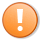# Quick-tip 25 | Changing Radarscreen cell background colors

A Gold Pass member asked how to go about creating a simple, example indicator that does the following:

Create an indicator for RadarScreen that calculate an 14 bar moving average (14MA). If the close of the symbol is above the 14MA then the RadarScreen would plot the word “ABOVE” in white with a green background.  If it is the first bar above the 14MA after a cross then the background color should be white with the word “ABOVE” plotted in green. In this way the user would know that it is the the first bar after a cross above the 14MA.This content is for members only.